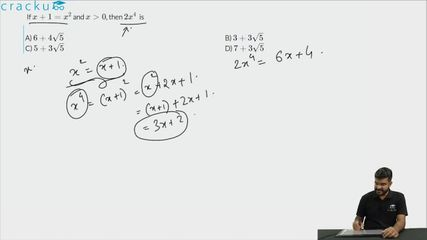Question 88

# If $$x+1=x^{2}$$ and $$x>0$$, then $$2x^{4}$$  is

Solution

We know that $$x^2 - x - 1=0$$
Therefore $$x^4 = (x+1)^2 = x^2+2x+1 = x+1 + 2x+1 = 3x+2$$
Therefore, $$2x^4 = 6x+4$$

We know that $$x>0$$ therefore, we can calculate the value of $$x$$ to be $$\frac{1+\sqrt{5}}{2}$$
Hence, $$2x^4 = 6x+4 = 3+3\sqrt{5}+4 = 3\sqrt{5}+7$$

### View Video Solution